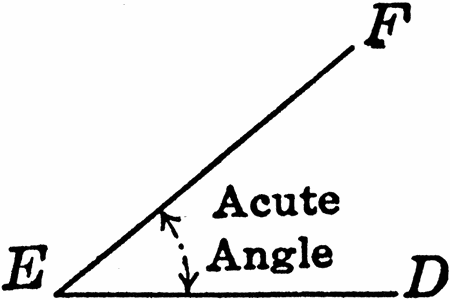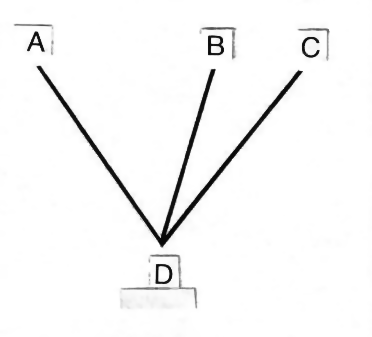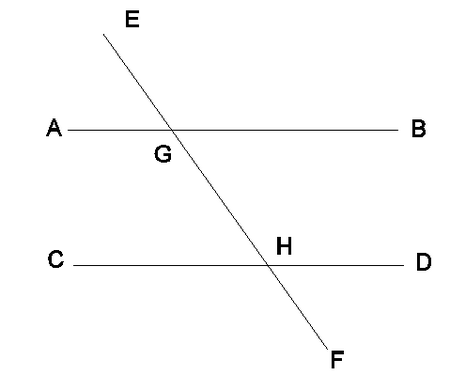## Types of Angles

Published on: Mar 21 2011 by Shenron

In geometry, an angle is defined as a space between two or more lines which are joined at a common point or space between two lines or surfaces that join to form a geometrical figure. In day to day life, angles play an important role. Angles come into act when making roads, designs, buildings, furniture and so on. Engineers, architects, carpenters all make use of angles in one way or another. Even artists use angles while sketching a painting or drawing.

When two lines join at a point, it gives rise to an angle. The point of intersection of lines is known as vertex. Angles are of different types. Let us have a look at some of the different types of angles.

Acute Angles

Acute angle is an angle which is less than 90 degrees.Right Angles

Right angle is an angle which is exactly 90 degrees in measure.

Obtuse Angles

Obtuse angle is angle who is more than 90 degrees and less than 180 degrees in measure. Any angle that measure between 90 degrees and 180 degrees will be an obtuse angle.

Straight Angles

Straight angle is an angle which is exactly 180 degrees in measure. It is pretty much similar to a straight line.

Reflex Angles

Reflex angle is an angle which is more than 180 degrees and less than 360 degrees in measure. All the angles that measure between 180 and 360 degrees are reflex angles.

Any angle that has a common vertex and a common side or edge usually forms two adjacent angles.Complementary Angles

Complementary angles are the angles where the measure of two angles gives you 90 degrees. It is important to note here that if two adjacent angles measure 90 degrees then they must be complementary angles rather than adjacent angles.

Supplementary Angles

When the measure of two angles gives you 180 degrees then these are supplementary angles.

Vertical Angles

Vertical angles are the angles with common vertex and having the sides formed from same lines. The given angles are vertical angles.

Similarly, if two parallel lines are crisscrossed by third line or transversal, you will have 8 angles formed in that case. Look at the given picture:

From these eight angles, four angles are interior angles and four are exterior angles.

Alternate Interior Angles

The angles which are formed between the two parallel lines because of transversal are interior angles.Alternate Exterior Angles

The angles which are formed on the outer side of the parallel lines along the transversal are called exterior angles.

Corresponding Angles

Corresponding angles are the angles which are formed in similar positions.